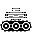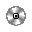Legacy GMboss arm rotation

phillipPbor

Member
I need help tho. i saw (little big planet has rotating arms from the wilderness level)
and i can be sure as well... how can you make a obj_boss move the obj_buzzsaw rotate back and forth around the OBJ_boss to protect it, while Obj_boss moving back and forth?

was there a coding?
Obj_bossObj_buzzsaw(is animating by frame so it don't need to rotate. just a path.

is there a path that made Obj_buzzsaw rotate the moving Obj_boss?

M

mikahon

Guest
You need trigonometry.
Code:
Obj_buzzsaw

- Creation event -
angle = 0;
distance = 128; //Set this to the distance between the two objects (in pixels)

- Step event -
angle += 1;//Set this for the rotation speed
x = tank.x+lengthdir_x(distance, angle);
y = tank.y+lengthdir_y(distance, angle);
Didn't test but should work.

Last edited by a moderator:

phillipPbor

Member
You need trigonometry.
Code:
Obj_buzzsaw

- Creation event -
angle = 0;
distance = 128; //Set this to the distance between the two objects (in pixels)

- Step event -
angle += 1;//Set this for the rotation speed
x = tank.x+lengthdir_x(distance, angle);
y = tank.y+lengthdir_y(distance, angle);
Didn't test but should work.
IT WORKED!
okay... so I get to know this fraction. so to make it go back and forth.
if the buzzsaw angle is on 180 then it will wait for 20 sec before going in reverse, if 0 then it will go forward until it reached 180.

do you think you can do a code like that?

NVM I GOT ITLast edited: